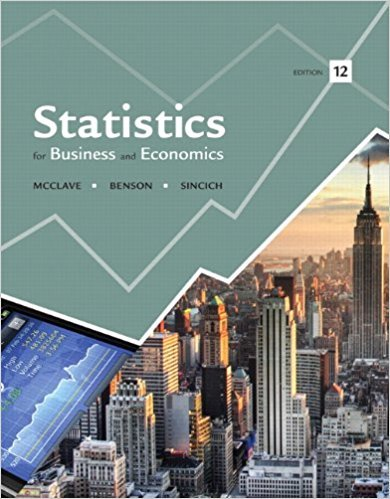×
Get Full Access to Statistics For Business And Economics - 12 Edition - Chapter 3 - Problem 5e
Get Full Access to Statistics For Business And Economics - 12 Edition - Chapter 3 - Problem 5e

×

# Compute the number of ways you can select n elements fromISBN: 9780321826237 51

## Solution for problem 5E Chapter 3

Statistics for Business and Economics | 12th Edition

• Textbook Solutions
• 2901 Step-by-step solutions solved by professors and subject experts
• Get 24/7 help from StudySoup virtual teaching assistantsStatistics for Business and Economics | 12th Edition

4 5 1 341 Reviews
19
5
Problem 5E

Problem 5E

Compute the number of ways you can select n elements from N elements for each of the following:

a. n = 2, N = 5

b. n = 3, N = 6

c. n = 5, N = 20

Step-by-Step Solution:

Solution:

Step 1 of 3:

1. The claim is to check the number of ways we can select n elements from N elements.

We have n = 2, and N = 5.

Let,==== 10

10 ways we can select n elements from N elements.

Step 2 of 3

Step 3 of 3

##### ISBN: 9780321826237

Unlock Textbook Solution